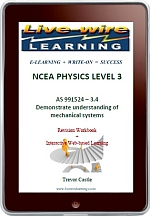### Physics AS 3.4 MechanicsThe contents include:
Impulse and Conservation of Momentum, Centre of Mass, Circular Motion, Resolving Forces in Circular Motion, Newton’s Law of Gravitation and Satellite Motion, Angular Velocity, Angular Acceleration, Torque and Rotational Inertia, Angular Momentum, Rotational Kinetic Energy, Simple Harmonic Motion: Reference Circle,  Simple Harmonic Motion: Energy, Simple Harmonic Motion: Graph and Phasor Representation.

Price: \$17.95
Quantity: